Courses

# Triangles - General Geometry Mathematics Notes | EduRev

## Mathematics : Triangles - General Geometry Mathematics Notes | EduRev

The document Triangles - General Geometry Mathematics Notes | EduRev is a part of the Mathematics Course Additional Topics for IIT JAM Mathematics.
All you need of Mathematics at this link: Mathematics

Congruent triangles
In Pre-algebra we learnt that triangles have three sides and three angles. We also learnt that the sum of the angles in a triangle is 180°.
If two triangles have the same size and shape they are called congruent triangles. If we flip, turn or rotate one of two congruent triangles they are still congruent.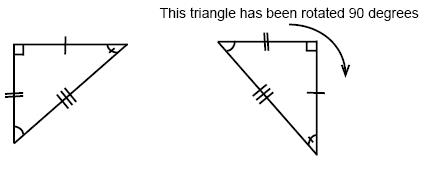If the sides of two triangles are the same then the triangles must have the same angles and therefore must be congruent. We can tell just from knowing the sides if two triangles are congruent or not.
If we know that two sides of a triangle and the angle between them are congruent to two sides and the angle between them of another triangle then these two triangles are also congruent.
Likewise if we know that two angles and the side between them are congruent to two angles and the side between them in another triangle then these two triangles are also congruent.
The last case that gives us congruent triangles is when two angles and a nonincluded side in one triangle are congruent to the corresponding angles and side of another triangle.

The circumcenter of a triangle is the point that is at an equidistance from the vertices of the triangle. In the following triangle, D is the circumcenter of the triangle and therefore are AD = BD = CD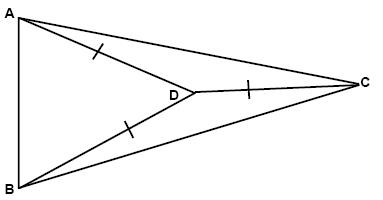If we split an angle in a triangle in the absolute middle then that gives us a bisector of that angle. Any point on the bisector is equidistant from the sides of the angle: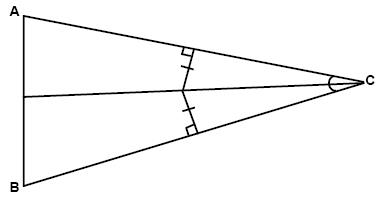The point that is equidistant to all sides of a triangle is called the incenter: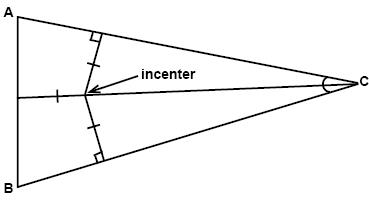A median is a line segment that has one of its endpoints in the vertex of a triangle and the other endpoint in the midpoint of the side opposite the vertex. The three medians of a triangle meet in the centroid. The centroid is located two thirds of the distance from any vertex of the triangle.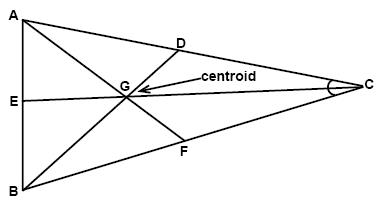Here EG = (2/3)EC, FG = (2/3)FA, and DG = (2/3)DB.
Every triangle has three altitudes. The altitude is a line segment that extends from a vertex and that is perpendicular to the side opposite the vertex. The intersection point of all three altitudes in a triangle is called the orthocenter: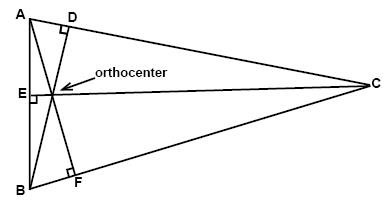Inequalities
The exterior angle of a triangle is always greater than either of its corresponding interior angles: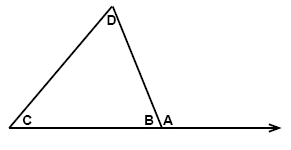m∠A > m∠C
m∠A > m∠D
Two important things to remember is that if one side of a triangle is longer than another side (compare BC and AB in the triangle below BC > AB) then the angle opposite of the longer side is always greater than the angle opposite (A > B) of the shorter side. Likewise if one angle is greater than another angle (A > B) then the side opposite of the greater angle is always longer than the side opposite the lesser angle (BC > AB).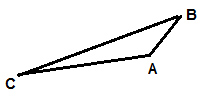The sum of the lengths of any two sides in a triangle is always greater than the length of the third side: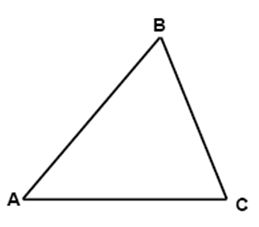AB + BC > AC
AC + AB > B
BC + AC > AB

Offer running on EduRev: Apply code STAYHOME200 to get INR 200 off on our premium plan EduRev Infinity!

40 docs

,

,

,

,

,

,

,

,

,

,

,

,

,

,

,

,

,

,

,

,

,

;